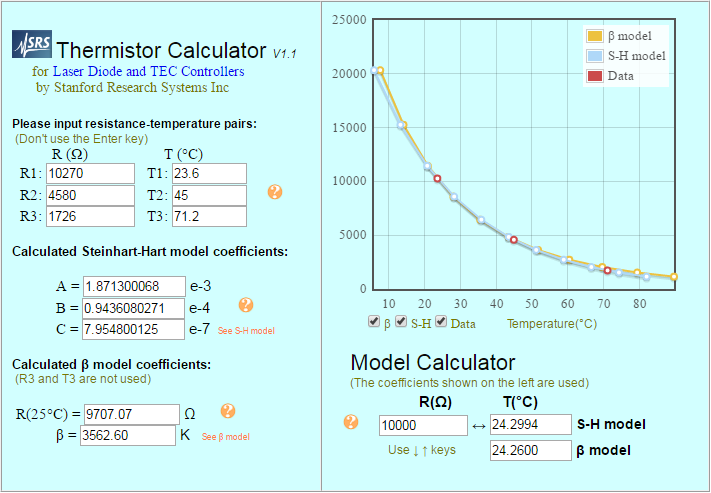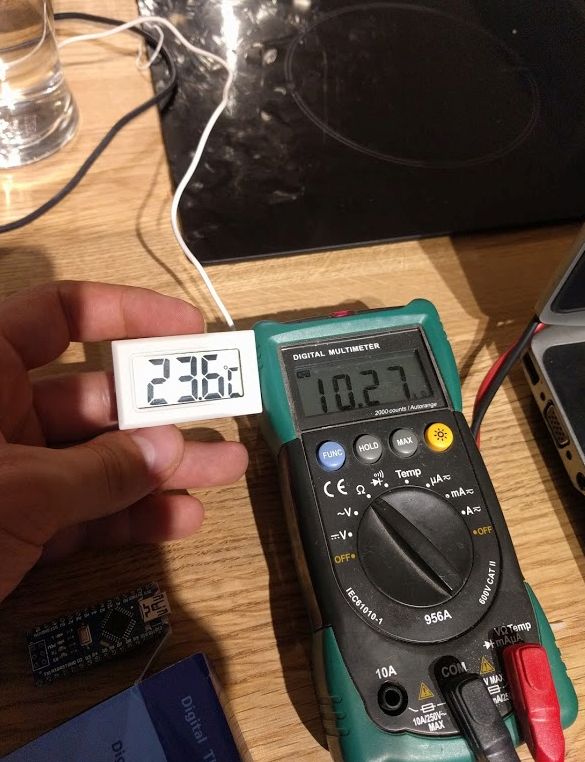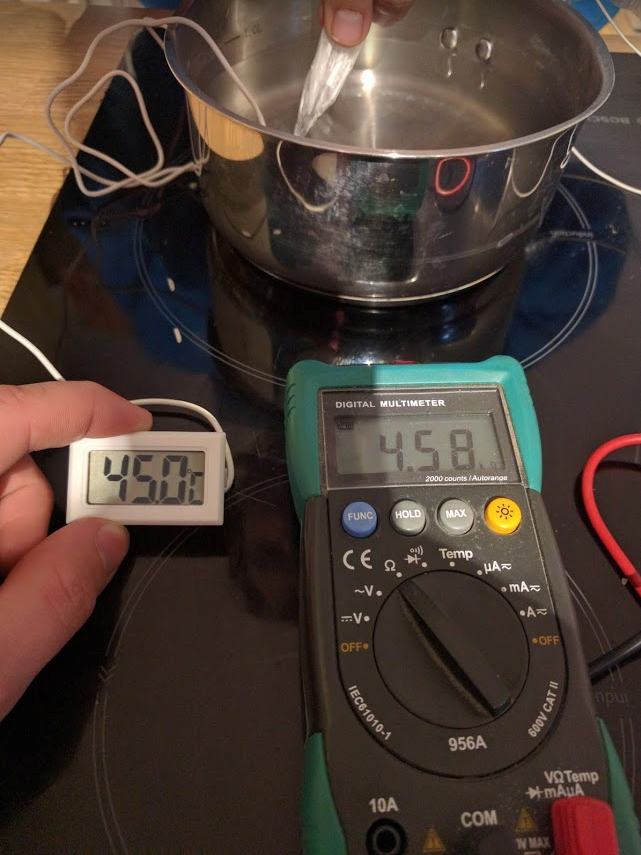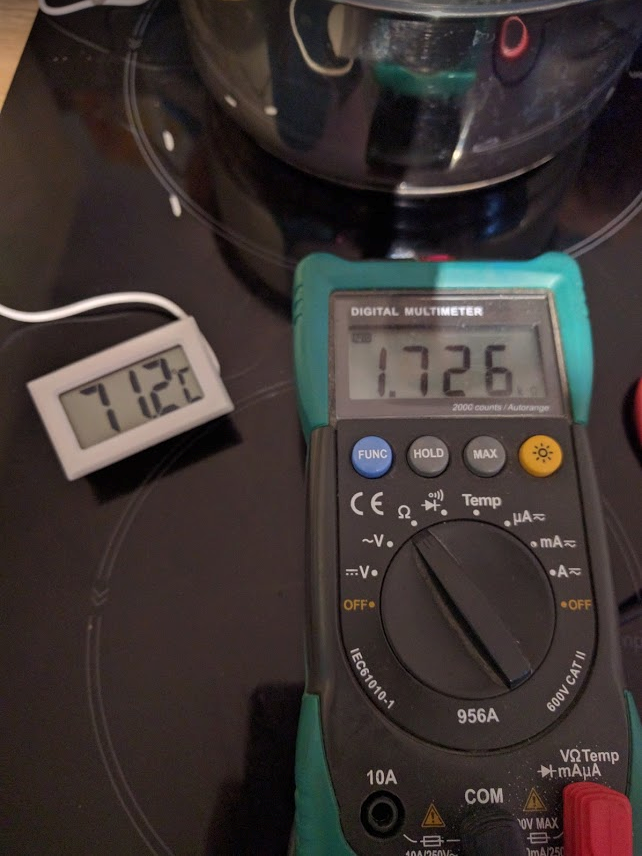# Temperature

These are the most involved sensors to calibrate, primarily due to the response of the thermistors being non-linear. A typical 10kΩ thermistor resistance vs temperature graph is shown below:Note that the 10k refers to the resistance at room temperature (25°), and that the resistance decreases as temperature increases. This is called a negative temperature coefficient, or NTC. The eChook board uses a 10k NTC Thermistor.
On the board, the thermistor completes the lower half of a potential divider circuit with a 10kΩ resistor, as such the voltage at the output of the potentiometer falls as the temperature of the thermistor increases.
To translate this voltage to a temperature, the resistance curve of the specific thermistor being used is needed. Due to tolerances, this is subtly different even between identical thermistors. To do this the eChook uses the Steinhart–Hart equation which uses three coefficients calculated from the thermistor to convert the resistance seen to an accurate temperature reading.
The first step in calibrating the thermistor is calculating these coefficients. Thankfully there’s a useful online calculator to help! All that is required is three measurements of the resistance of the thermistor at different, known temperatures. The calculator can be found here and looks like this:Enter the three temperature and resistance measurements and copy the A, B and C coefficients from the calculator into the calibration.h file.
//Board and Sensor Specific Calibrations
const float CAL_THERM_A = 0.001871300068; //Steinhart-Hart constants - See documentation for calibration method
const float CAL_THERM_B = 0.00009436080271;
const float CAL_THERM_C = 0.0000007954800125;
To get the default calibration we used a pan of water, digital thermometer and a multimeter. We wrapped the thermistor in cling film to prevent the water conducting across the legs and altering the reading.